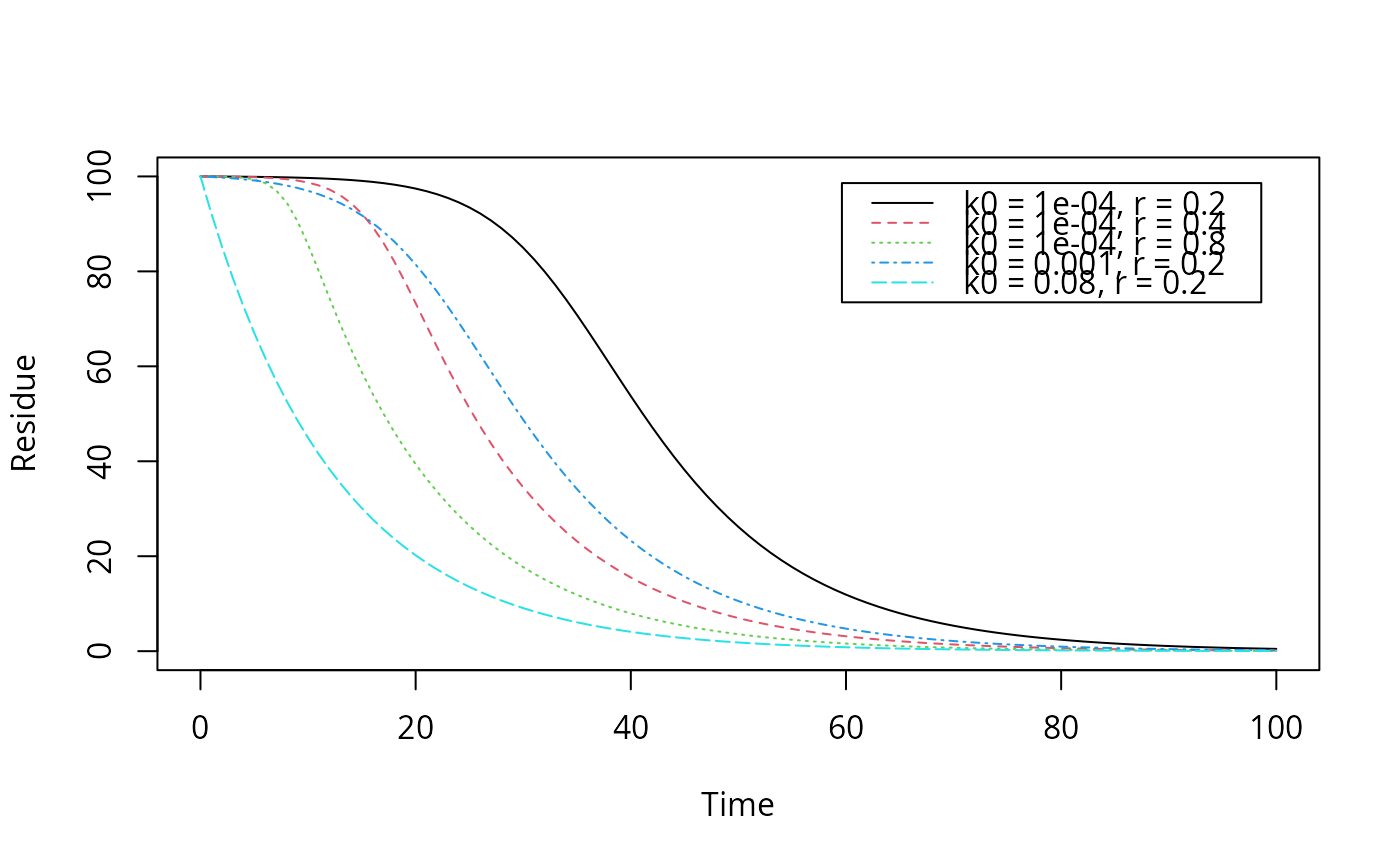Function describing exponential decline from a defined starting value, with an increasing rate constant, supposedly caused by microbial growth

logistic.solution(t, parent_0, kmax, k0, r)

## Arguments

t

Time.

parent_0

Starting value for the response variable at time zero.

kmax

Maximum rate constant.

k0

Minimum rate constant effective at time zero.

r

Growth rate of the increase in the rate constant.

## Value

The value of the response variable at time t.

## Note

The solution of the logistic model reduces to the SFO.solution if k0 is equal to kmax.

Other parent solutions: DFOP.solution(), FOMC.solution(), HS.solution(), IORE.solution(), SFO.solution(), SFORB.solution()

## Examples


# Reproduce the plot on page 57 of FOCUS (2014)
plot(function(x) logistic.solution(x, 100, 0.08, 0.0001, 0.2),
from = 0, to = 100, ylim = c(0, 100),
xlab = "Time", ylab = "Residue")
plot(function(x) logistic.solution(x, 100, 0.08, 0.0001, 0.4),
from = 0, to = 100, add = TRUE, lty = 2, col = 2)
plot(function(x) logistic.solution(x, 100, 0.08, 0.0001, 0.8),
from = 0, to = 100, add = TRUE, lty = 3, col = 3)
plot(function(x) logistic.solution(x, 100, 0.08, 0.001, 0.2),
from = 0, to = 100, add = TRUE, lty = 4, col = 4)
plot(function(x) logistic.solution(x, 100, 0.08, 0.08, 0.2),
from = 0, to = 100, add = TRUE, lty = 5, col = 5)
legend("topright", inset = 0.05,
legend = paste0("k0 = ", c(0.0001, 0.0001, 0.0001, 0.001, 0.08),
", r = ", c(0.2, 0.4, 0.8, 0.2, 0.2)),
lty = 1:5, col = 1:5)# Fit with synthetic data
logistic <- mkinmod(parent = mkinsub("logistic"))

sampling_times = c(0, 1, 3, 7, 14, 28, 60, 90, 120)
parms_logistic <- c(kmax = 0.08, k0 = 0.0001, r = 0.2)
d_logistic <- mkinpredict(logistic,
parms_logistic, c(parent = 100),
sampling_times)summary(m)$bpar #> Estimate se_notrans t value Pr(>t) Lower #> parent_0 1.057896e+02 1.9023449649 55.610120 3.768361e-16 1.016451e+02 #> kmax 6.398190e-02 0.0143201029 4.467978 3.841828e-04 3.929235e-02 #> k0 1.612775e-04 0.0005866813 0.274898 3.940351e-01 5.846685e-08 #> r 2.263946e-01 0.1718110773 1.317695 1.061044e-01 4.335843e-02 #> sigma 5.332935e+00 0.9145907310 5.830952 4.036926e-05 3.340213e+00 #> Upper #> parent_0 109.9341588 #> kmax 0.1041853 #> k0 0.4448750 #> r 1.1821121 #> sigma 7.3256566 endpoints(m)$distimes